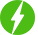# 2023 Python for Linear Regression in Machine Learning

Deal Score0
Free \$19.99
Deal Score0
Free \$19.99

2023 Python for Linear Regression in Machine Learning, Linear & Non-Linear Regression, Lasso & Ridge Regression, SHAP, LIME, Yellowbrick, Feature Selection & Outliers Removal.

Unlock the power of machine learning with our comprehensive Python course on linear regression. Learn how to use Python to analyze data and build predictive models. This course is perfect for beginners with little or no programming experience and experienced Python developers looking to expand their skill set.

You’ll start with the basics of Python and work your way up to advanced techniques like linear regression, which is a powerful tool for predicting future outcomes based on historical data. Along the way, you’ll gain hands-on experience with popular Python libraries such as NumPy, Pandas, and Matplotlib. We will also cover the important aspect of model optimization, interpretability, and feature selection. You will learn how to optimize your model to improve its performance and how to interpret the model results and understand the underlying relationships in your data. We will also discuss feature selection techniques that are used to identify the most essential features that drive the predictions.

By the end of the course, you’ll have a solid understanding of how to use Python to build linear regression models and make accurate predictions. You’ll also be able to apply your new skills to a wide range of machine learning and data science projects. So, if you’re ready to take your Python skills to the next level and start using machine learning to analyze and predict real-world outcomes, this is the course for you!

What is covered in this course?

This course teaches you, step-by-step coding for Linear Regression in Python. The Linear Regression model is one of the widely used in machine learning and it is one the simplest ones, yet there is so much depth that we are going to explore in 14+ hours of videos.

Below are the course contents of this course:

• Section 1- IntroductionThis section gets you to get started with the setup. Download resources files for code along.
• Section 2- Python Crash CourseThis section introduces you to the basics of Python programming.
• Section 3- Numpy IntroductionThis section is optional, you may skip it but I would recommend you to watch it if you are not comfortable with NumPy.
• Section 4- Pandas IntroductionThis section introduces you to the basic concepts of Pandas. It will help you later in the course to catch up on the coding.
• Section 5- Matplotlib IntroductionDo not skip this section. We will be using matplotlib plots extensively in the coming sections. It builds a foundation for a strong visualization of linear regression results.
• Section 6- Linear Regression IntroductionWe will kick-start our Linear Regression learning. You will learn the basics of linear regression. You will see some examples so that you can understand how Linear Regression works and how to analyze the results.
• Section 7- Data Preprocessing for Linear RegressionThis section is the most important section. DO NOT SKIP IT. It builds the foundation of data preprocessing for linear regression and other linear machine learning models. You will be learning, what are the techniques which we can use to improve the performance of the model. You will also learn how to check if your data is satisfying the coding of Linear Model Assumptions.
• Section 8- Machine Learning Models Interpretability and ExplainerThis section teaches you how to open-up any machine learning models. Now you don’t need to treat machine learning models as black-box, you will get to learn how to open this box and how to analyze each and every component of machine learning models.
• Section 9- Linear Regression Model OptimizationThis section extensively uses the knowledge of previous sections so don’t skip those. You will learn various techniques to improve model performance. We will show you how to do outliers removal and feature transformations.
• Section 10- Feature Selection for Linear RegressionThis section teaches you some of the best techniques of feature selection. Feature selection reduces the model complexity and chances of model overfitting. Sometimes the model also gets trained faster but mostly depends on how many features are selected and the types of machine learning models.
• Section 11- Ridge & Lasso Regression, ElasticNet, and Nonlinear RegressionThis section covers, various types of regression techniques. You will be seeing how to achieve the best accuracy by using the above techniques.

By the end of this course, your confidence will boost in creating and analyzing the Linear Regression model in Python. You’ll have a thorough understanding of how to use regression modeling to create predictive models and solve real-world business problems.

We will be happy to hear your thoughts• Total (0)
0